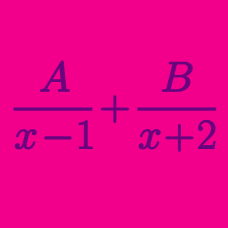Algebra

# Partial Fractions: Level 4 Challenges

$\frac {1}{1 \times 2} + \frac {1}{1 \times 5} + \frac {1}{3 \times 3} + \frac {1}{2 \times 7} + \frac {1}{5 \times 4} + \ldots$

Find the sum of this infinite series.

$S = \frac {1}{1\times 2 \times 3} + \frac {1}{2\times 3 \times 4} + \ldots + \frac {1} {(n)(n+1)(n+2)}$ $+ \ldots +\frac {1}{14 \times (14+1) \times (14+2)} = \frac {a} {b},$ where $a$ and $b$ are positive, coprime integers. What is the value of $a + b$?

$\displaystyle{\sum _{ n=1 }^{ 2015 }{ \frac { { n }^{ 2 }+n+1 }{ \left( { n }^{ 2 }+n \right) \left( n+1 \right) ! } }}$ can be represented in the form $\dfrac { a }{ b }$, where $a$ and $b$ are coprime positive integers. Find the value of $a-b$.

$\displaystyle\sum_{n=0}^{\infty} \dfrac{1}{2015^{2^{n}} - 2015^{-(2^{n})}}$

If the closed form of the series above is in the form $\frac a b$, where $a$ and $b$ are positive coprime integers, then find $b - a.$

For each positive integer $n$, let $H_n = \frac{1}{1} + \frac{1}{2} + \cdots + \frac{1}{n}.$ If $\sum_{n=4}^{\infty} \frac{1}{nH_nH_{n-1}} = \frac{a}{b}$ for relatively prime positive integers $a$ and $b$, find $a+b$.

This problem is shared by Sandeep S.

×